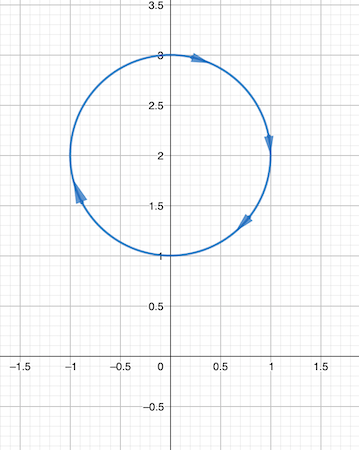# Sketch the parameterized curve in the plane \begin{cases} x(t) = \sin t \\ y(t) = 2+ \cos t \end...

## Question:

Sketch the parameterized curve in the plane {eq}\begin{cases} x(t) = \sin t \\ y(t) = 2+ \cos t \end {cases} \ t \in [0,2\pi] {/eq}

## Parametric Curves

A curve given parametrically as {eq}\displaystyle x=f(t), y=g(t), a\leq t\leq b, {/eq} is a curve whose points {eq}\displaystyle (x,y), {/eq} are obtained by giving values to {eq}\displaystyle t. {/eq}

The direction of a parametric curve is given by the direction of increasing {eq}\displaystyle t. {/eq}

Sometimes, to plot a parametric curve, we convert the curve in Cartesian form, by eliminating the parameter {eq}\displaystyle t, {/eq} and obtaining a relationship between {eq}\displaystyle x\text{ and } y. {/eq}

If there are sine and cosine function terms, we will use trigonometric identities to eliminate the parameter, like the fundamental identity in trigonometry {eq}\displaystyle \sin^2 t+\cos^2 t=1. {/eq}

To sketch the graph of the parametric curve {eq}\displaystyle x=\sin t, y=2+\cos t, 0\leq t\leq 2\pi {/eq} we will obtain the Cartesian form to identify the curve.

{eq}\displaystyle x=\sin t, y=2+\cos t\iff \sin t=x, \cos t=y-2\implies \sin^2 t+\cos^2 t=1=x^2+(y-2)^2, {/eq}

so the curve is a circle centered at {eq}\displaystyle (0,2) {/eq} and radius 1.

Because a parametric curve has a direction, given by tracing the curve for increasing values of {eq}\displaystyle t, {/eq} the direction of the curve is from {eq}\displaystyle (0,3) \text{ for }t=0 \text{ to } (1,2) \text{ for }t=\frac{\pi}{2}, {/eq} which is a clockwise direction.

The graph of the parametric curve is shown below by the blue solid line.